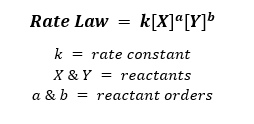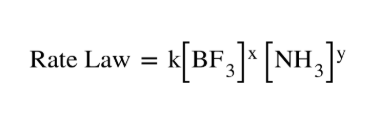# Problem: BF3 (g) + NH3(g) F3BNH3 (g)For the above reaction, the following data were measured:Experiment [BF3]  (M)  [NH3]  (M) Initial Rate (M/s) 10.2500.2500.213020.2500.1250.106530.2000.1000.068240.3500.1000.119350.1750.1000.0596What is the overall order of the reaction?

###### FREE Expert Solution
• Overall order can be calculated by determining the sum of orders of each reactant.

• Rate law relies on the concentrations of the reactants where:• Rate law for the reaction will appear as:• For the reactant order, we must look for the data where the concentration of one changes while the other remains constant.

• Calculating order with respect to NH3###### Problem Details

BF3 (g) + NH3(g) F3BNH3 (g)

For the above reaction, the following data were measured:

 Experiment [BF3]  (M) [NH3]  (M) Initial Rate (M/s) 1 0.250 0.250 0.2130 2 0.250 0.125 0.1065 3 0.200 0.100 0.0682 4 0.350 0.100 0.1193 5 0.175 0.100 0.0596

What is the overall order of the reaction?